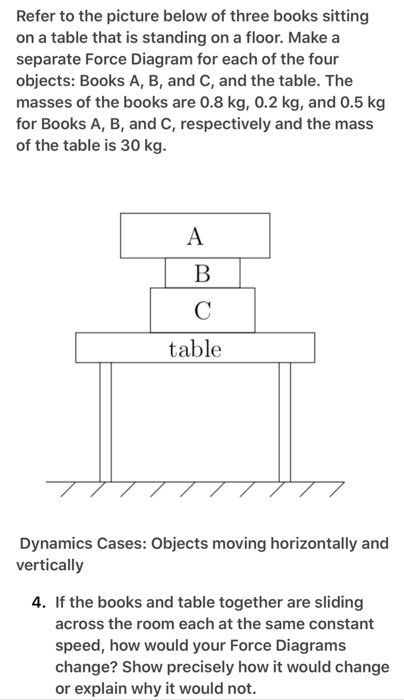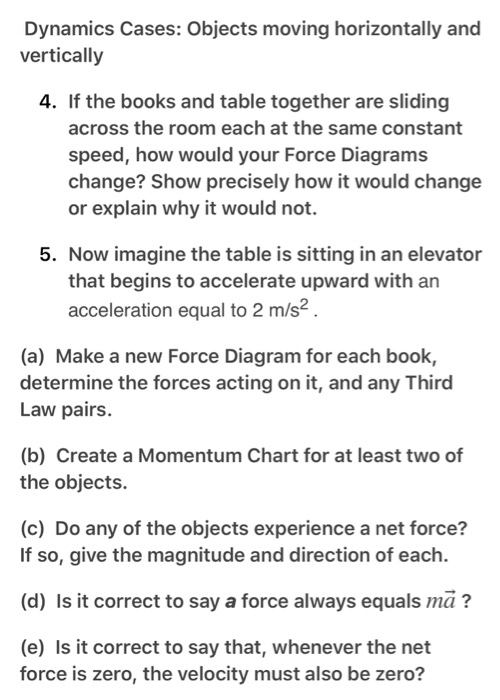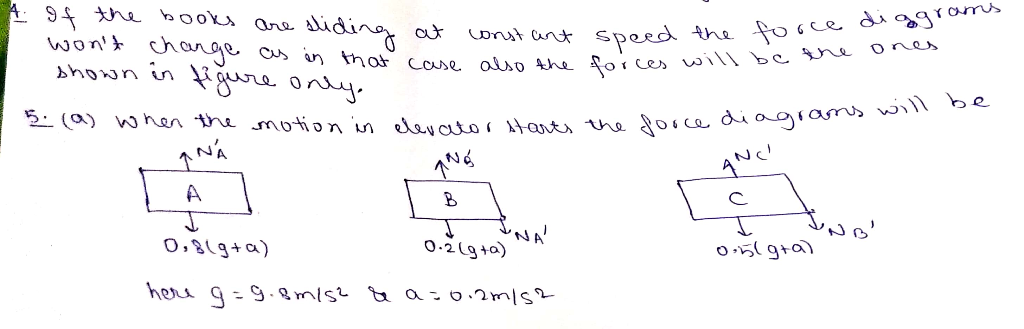Homework Help Question & Answers

Refer to the picture below of three books sitting on a table that is standing on a floor. Make a ...Refer to the picture below of three books sitting on a table that is standing on a floor. Make a separate Force Diagram for each of the four objects: Books A, B, and C, and the table. The masses of the books are 0.8 kg, 0.2 kg, and 0.5 kg for Books A, B, and C, respectively and the mass of the table is 30 kg. table Dynamics Cases: Objects moving horizontally and vertically 4. If the books and table together are sliding across the room each at the same constant speed, how would your Force Diagrams change? Show precisely how it would change or explain why it would not
Dynamics Cases: Objects moving horizontally and vertically 4. If the books and table together are sliding across the room each at the same constant speed, how would your Force Diagrams change? Show precisely how it would change or explain why it would not. 5. Now imagine the table is sitting in an elevator that begins to accelerate upward with an acceleration equal to 2 m/s2 (a) Make a new Force Diagram for each book, determine the forces acting on it, and any Third Law pairs (b) Create a Momentum Chart for at least two of the objects. (c) Do any of the objects experience a net force? If so, give the magnitude and direction of each. (d) Is it correct to say a force always equals mã? (e) Is it correct to say that, whenever the net force is zero, the velocity must also be zero?please rate it up. Thanks

Add Answer of: Refer to the picture below of three books sitting on a table that is standing on a floor. Make a ...
More Homework Help Questions Additional questions in this topic.

• sam and sylvia work at a book store , duing a sale sam sold 15% more books than sylvia If sam sold 69 books how many did sylvia sell is it 54 books

Need Online Homework Help?

Get FREE EXPERT Answers
WITHIN MINUTES
Related Questions